# NCERT Solutions Class 9 Maths Chapter 5 INTRODUCTION TO EUCLIDS GEOMETRY

Ncert Solutions for class 9 subject Maths Chapter 5 INTRODUCTION TO EUCLIDS GEOMETRYin pdf Best Free NCERT Solutions for class 1 to 12 in pdf NCERT Solutions, cbse board, Maths, ncert Solutions for Class 9 Maths, class 9 Maths ncert solutions, INTRODUCTION TO EUCLIDS GEOMETRY, Class 9, ncert solutions chapter 5 INTRODUCTION TO EUCLIDS GEOMETRY, class 9 Maths, class 9 Maths ncert solutions, Maths ncert solutions class 9, Ncert Solutions Class 9 Mathematics Chapter 5 INTRODUCTION TO EUCLIDS GEOMETRY

## Concept Chapter 5 Class 9 Maths INTRODUCTION TO EUCLIDS GEOMETRY Ncert Solutions

1. Though Euclid defined a point, a line, and a plane, the definitions are not accepted by mathematicians. Therefore, these terms are now taken as undefined.
2. Axioms or postulates are the assumptions which are obvious universal truths. They are not proved.
3. Theorems are statements which are proved, using definitions, axioms, previously proved statements and deductive reasoning.

Some of Euclid’s axioms were:
(1) Things which are equal to the same thing are equal to one another.
(2) If equals are added to equals, the wholes are equal.
(3) If equals are subtracted from equals, the remainders are equal.
(4) Things which coincide with one another are equal to one another.
(5) The whole is greater than the part.
(6) Things which are double of the same things are equal to one another.
(7) Things which are halves of the same things are equal to one another.

Definitions
1. A point is that which has no part.
2. A line is breathless length.
3. The ends of a line are points.
4. A straight line is a line which lies evenly with the points on itself.
5. A surface is that which has length and breadth only.
6. The edges of a surface are lines.
7. A plane surface is a surface which lies evenly with the straight lines on itself.
5.

Euclid’s postulates were :
Postulate 1 : A straight line may be drawn from any one point to any other point.
Axiom 5.1 : Given two distinct points, there is a unique line that passes through them.
How many lines passing through P also pass through Q (see Fig. 5.4)? Only one, that is, the line PQ. How many lines passing through Q also pass through P? Only one, that is, the line PQ. Thus, the statement above is self-evident, and so is taken as an axiom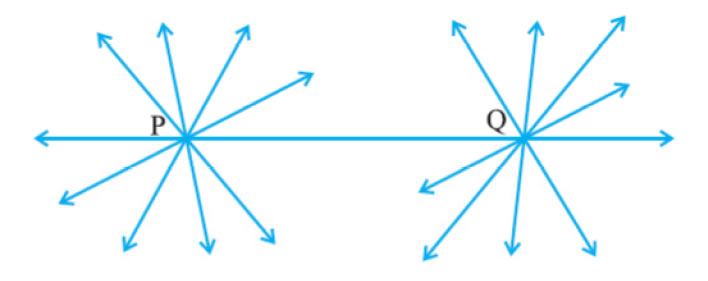Postulate 2 : A terminated line can be produced indefinitely.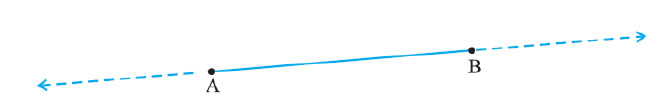Postulate 3 : A circle can be drawn with any centre and any radius.
Postulate 4 : All right angles are equal to one another.
Postulate 5 : If a straight line falling on two straight lines makes the interior angles on the same side of it taken together less than two right angles, then the two straight lines, if produced indefinitely, meet on that side on which the sum of angles is less than two right angles.

Two equivalent versions of Euclid’s fifth postulate are:
(i) ‘For every line l and for every point P not lying on l, there exists a unique line m
passing through P and parallel to l’.
(ii) Two distinct intersecting lines cannot be parallel to the same line. All the attempts to prove Euclid’s fifth postulate using the first 4 postulates failed. Butthey led to the discovery of several other geometries, called non-Euclideangeometries.
Theorem 5.1 : Two distinct lines cannot have more than one point in common.
Proof : Here we are given two lines l and m. We need to prove that they have only onepoint in common.
For the time being, let us suppose that the two lines intersect in two distinct points, sayP and Q. So, you have two lines passing through two distinct points P and Q. But thisassumption clashes with the axiom that only one line can pass through two distinctpoints. So, the assumption that we started with, that two lines can pass through twodistinct points is wrong.
We are forced to conclude that two distinct lines cannot have more than one point in common

## Exercise 5.1 Chapter 5 Class 9 Maths INTRODUCTION TO EUCLIDS GEOMETRY Ncert Solutions

1. Which of the following statements are true and which are false? Give reasons for your answers.
(i) Only one line can pass through a single point.
(ii) There are an infinite number of lines which pass through two distinct points.
(iii) A terminated line can be produced indefinitely on both the sides.
(iv) If two circles are equal, then their radii are equal.
(v) In the following figure, if AB = PQ and PQ = XY, then AB = XY.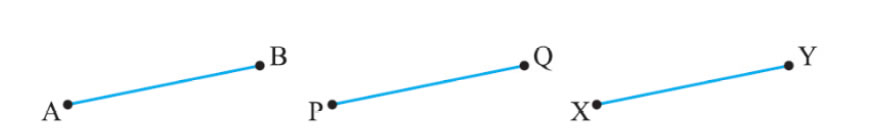(i)False.

Correct statement
Through a single point, infinite number of lines can pass.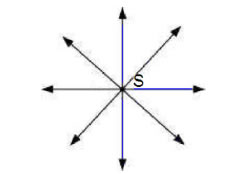In above figure, you can see that there are infinite numbers of lines can pass through a single point S.

(ii) False.
Correct statement
through two distinct points, only one line can pass.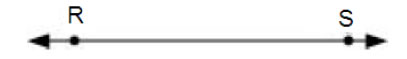In above figure, it can see that there is only one single line that drawn through two
Distinct points R and S.
(iii) True.
According to Euclid’s laws, A terminated line can be produced indefinitely on both the sides.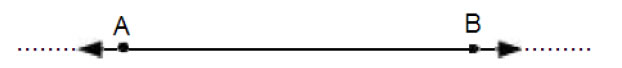In above figure, you can see there are indefinitely lines both side

(iv)True.
According to axioms 4 of the Euclid, If two circles are equal, then their centre and circumference will coincide and hence, the radii will also be equal.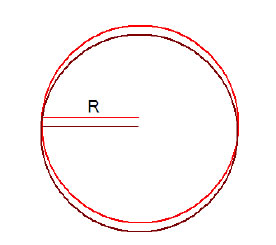In above figure, it can see both circle have equal radius

(v) True.
According to Euclid’s axiom first, things which are equal to the same thing,

And in our given problem it is given that AB and XY are two terminated lines and both are equal to a third line PQ are equal to one another. Therefore, the lines AB and XY will be equal to each other.

2. Give a definition for each of the following terms. Are there other terms that need to be defined first? What are they, and how might you define them?
(i) parallel lines (ii) perpendicular lines (iii) line segment
(iv) radius of a circle (v) square
Solution.
(i) parallel lines

(1) Parallel straight lines are straight lines which, being in the same plane and being produced indefinitely in both directions.
(2) Perpendicular distance between two lines is always constant
(3) Parallel lines do not meet one another in either direction.
(4) These lines never intersect each other.
(5) in below figure R and S are parallel lines and K is the perpendicular distance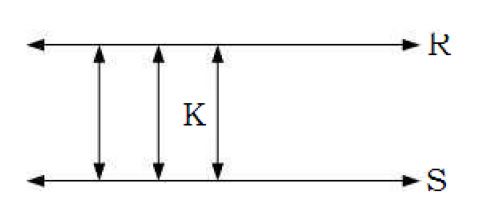Perpendicular lines
These lines always intersect one another at right angle (90 degree)
In below figure P and Q are perpendicular lines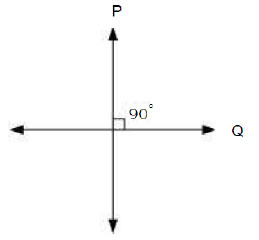(iii) Line segment.
A straight line drawn from any point to any other point is called as line segment.
A line segment which extends indefinitely in both directions gives a line.

Here PQ is a line segment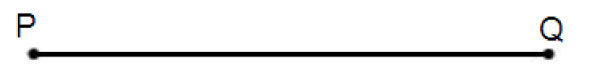A line segment joining the centre to any point on the circle is called the radius of the circle. It is the distance between the center of a circle to any point lying on the circle.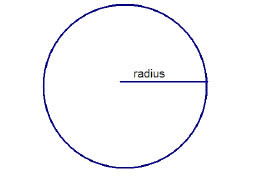(v) Square.
A square is that which is both equilateral and right-angled. That means all sides are equal and each angle is 90 degree.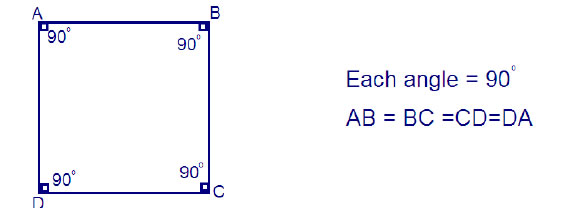3. Consider two ‘postulates’ given below:
(i) Given any two distinct points A and B, there exists a third point C which is in between A and B.
(ii) There exist at least three points that are not on the same line.
Do these postulates contain any undefined terms? Are these postulates consistent?
Do they follow from Euclid’s postulates? Explain.

Solutions :-
There are several undefined terms in the given postulates.
The given postulates are consistent because they refer to two different situations.
In first postulate two points A and B are given points then there will point C lying in the line between them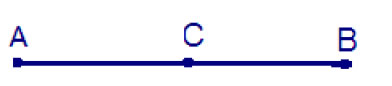Postulate (ii) states that for the given points A and B we can take point C not lying on the lines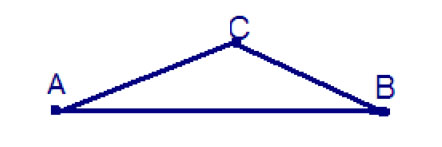These postulates do not follow from Euclid’s postulates. However they follow from the axiom, given two distinct points, there is a unique line that passes through them”.

4. If a point C lies between two points A and B such that AC = BC, then prove that AC =1/2AB. Explain by drawing the figure.
Solution

Given that C is the lies between both points A and B
And
AC = BC
Add AC both side we get
AC + AC = BC + AC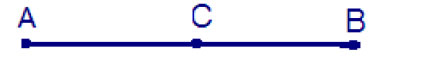According to definition of Euclid , if equals are added to equals, whole will equal
Here, (BC + AC) coincides with AB. It is known that things which coincide with one another are equal to one another.
2AC = AB
Divide by 2 we get
AC = AB/2
Hence proved

5. In Question 4, point C is called a mid-point of line segment AB. Prove that every line segment has one and only one mid-point.
Let C and D are two midpoints of the line segments AB
According to Euclid’s axioms 4
AC = BC ...(1)
D is also a mid point so that
We have AB = AB … (3)
And we know AB = AC + CB
From equation (iii)
AC + CB = AD + DB
From equation (1) and (2)plug the value of BC and DB we get
Divide by 2 we get
Both points are on same line so both points will superimpose and D and C are exactly at the same place
Hence midpoint of the lines segment is always unique

6. In Fig. 5.10, if AC = BD, then prove that AB = CD.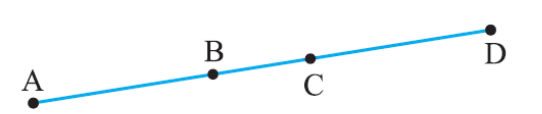From the above figure we get that
AC = AB + BC
BD = BC + CD
It is given that AC = BD

Plug the value of AC we get
AB + BC = BC + CD … (1)
According to Euclid’s axiom,when equals are subtracted from equals, the remainders
are also equal.
Subtracting BC from both side in equation (1), we get
AB + BC − BC = BC + CD − BC
AB = CD
Hence proved

7. Why is Axiom 5, in the list of Euclid’s axioms, considered a ‘universal truth’? (Note that the question is not about the fifth postulate.)

Euclid's Axiom 5 states that "The whole is greater than the part."
Since this is true for anything in any part of the world.
So, this is a universal truth.

## Exercise 5.2 Chapter 5 Class 9 Maths INTRODUCTION TO EUCLIDS GEOMETRY Ncert Solutions

1. How would you rewrite Euclid’s fifth postulate so that it would be easier to understand?

Solution.
There are several easy equivalent versions of Euclid's fifth postulate.
Two lines are said to be parallel if they are equidistant from one other and they do not have any point of intersection.
Then, by Playfair’s axiom (equivalent to the fifth postulate), there is a unique line m through P which is parallel to l.

2. Does Euclid’s fifth postulate imply the existence of parallel lines? Explain

Yes. Euclid's fifth postulate is imply for parallelism of lines because if a straight line l falls on two straight lines m and n such that sum of the interior angles on one side of l is two right angles, then by Euclid's fifth postulate the line will not meet on this side of l.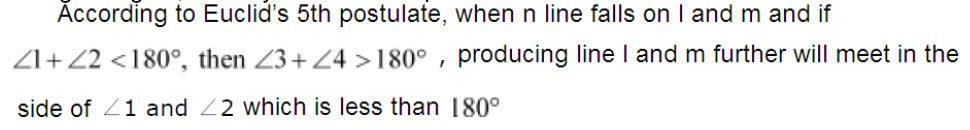### NCERT Books Free Pdf Download for Class 5, 6, 7, 8, 9, 10 , 11, 12 Hindi and English Medium

 Mathematics Biology Psychology Chemistry English Economics Sociology Hindi Business Studies Geography Science Political Science Statistics Physics Accountancy